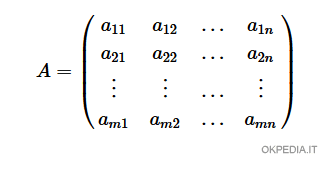# How to make a matrix in Matlab or Octave

To create a matrix in Matlab (or Octave) type this command

matrix = [ a11,a12,a13, ... ; a21, a22, a23, ... ]

The elements of a row of the matrix ( a11, a12, a13, ... ) are separated from each other by a comma (,).

Each row of the matrix is separated from the next row by the semicolon symbol (;)## Examples

Example 1 (square matrix)

To define the 2x2 square matrix

$$\begin{pmatrix} 1 & 2 \\ 3 & 4 \end{pmatrix}$$

The array is assigned to a variable M

M = [ 1,2 ; 3, 4 ]

The matrix has two rows and two columns.

Example 1 (rectangular matrix)

Define a 2x3 rectangular matrix

$$\begin{pmatrix} 1 & 2 & 3 \\ 4 & 5 & 6 \end{pmatrix}$$

The array is assigned to a variable M

M = [ 1,2,3 ; 4,5,6 ]

In this case there are three columns and two rows.

Example 3 (vector)

To create a vector

$$\begin{pmatrix} 1 \\ 2 \\ 3 \end{pmatrix}$$

The vector is assigned to a variable v

v = [ 1;2;3 ]

The column matrix (vector) has a single column and three rows.

https://how.okpedia.org/en/matlab/how-to-make-a-matrix-in-matlab-or-octaveReport us an error or send a suggestion to improve this page

Matrix in Matlab/Octave